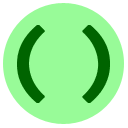# 7C: Loops

Lesson 7 has three parts A, B, C which can be completed in any order.

So far we have learned basic programming commands (e.g. assigning values, printing) and, a way to control which statements are executed using the `if` statement. In this lesson, we introduce loops (sometimes called repetition or iteration): a way to make a computer do the same thing (or similar things) over and over. For example, spell-checking every word in a document would be done with a loop. We will describe the two kinds of Python loops in this lesson: `while` loops and `for` loops.

## `while` Loops

A `while` statement repeats a section of code over and over again as long as some condition is true. Here is an example:

Here is the general structure:

• The first line is `while «condition»:` where «condition» is an expression which returns `True` or `False` (a Boolean expression, like with `if` statements).
• Afterwards, we put an indented block (again, like an `if` statement) consisting of the statements which we want to be repeated over and over. This is called the body.
• When you run the program, the following is repeated:
• The condition is tested; if the condition is `True` then the body is executed and afterwards we repeat from the top; once the condition is evaluated to `False`, the loop stops.

So in the example above, we keep repeating the loop body until `timeLeft` is not greater than 0.Coding Exercise: Countup
Modify the example above to give a program where we count up starting from 1 and going up until 10, and then print `Blastoff!`
You may enter input for the program in the box below.With loops, it is easy to write a program that runs forever in an infinite loop.
Example
Infinite loop
You get the error "Time Limit Exceeded" because the CS Circles web server enforces a time limit; after 1 second the program is terminated. If you ran the program at home, it would run forever (until you yourself force it to terminate, usually by pressing Ctrl-C).

## `for` Loops

There is another kind of loop in Python called a `for` loop. In many situations either kind of loop (`for`/`while`) can be used but one is simpler than another, so it is useful to know how to use both. A `for` loop is built in order to easily loop through a range of numbers (or as we will see in a later lesson, any list of data).

Here is an example of a `for` loop.

The general structure of a numerical `for` loop is

```for «variableName» in range(«startValue», «tailValue»):
«indented block of commands, called the loop "body"»```
As usual, the body block can be multiple lines long, as long as all of those lines are indented by the same amount. First the loop body is executed with `variableName` set to `startValue.` Then it repeats with `variableName` set to `startValue+1`, then again with `startValue+2`, et cetera. This continues until `variableName` has value `tailValue-1`, and afterwards the loop stops.The loop ends with `tailValue-1`, and not `tailValue`!
Example

Here is an example of a `for` loop inside another `for` loop.

Example
This code prints a 5×5 square of ones.
Note: when we multiply a number `X` by ten and add one, we're essentially putting an extra `1` digit at the end of `X`. For example, (1867*10)+1=18671.Coding Exercise: One Triangle
Modify the previous program in two ways. First, instead of a square, make it draw a triangle shaped like this: ◤. Second, instead of always having 5 lines, it should take the desired size as input from `input()`. For example, if the input is `3`, then the output should be

`111111`
You may enter input for the program in the box below.

## The `break` and `continue` Statements

The `break` statement is like an emergency escape for a `while` or `for` loop: `break` causes an immediate jump to the commands after the end of the loop body. Here is an example using `break`: it reads all lines of input until it finds one that says `"END"`.

Example
Looping through all lines of input

The `continue` statement makes you skip the rest of a loop, then repeat the body from the next round (usually called the next "iteration").

Example

Here is a visualized example that combines `break` and `continue`. Can you predict what it will output?

## ExercisesCoding Exercise: Square Census
The square numbers are the integers of the form K × K, e.g. 9 is a square number since 3 × 3 = 9. Write a program that reads an integer n from input and outputs all the positive square numbers less than n, one per line in increasing order. For example, if the input is 16, then the correct output would be

`149`
Hint
You may enter input for the program in the box below.Coding Exercise: Skipping
Extend the "Looping through all lines of input" example above (we've copied it for you) by adding a new feature: any line equal to `SKIP` should not be printed, and should not cause the counter to be increased. Run the program to see an example. Hint
You may enter input for the program in the box below.Coding Exercise: Finding Factors
If a × b = n, we call a × b a factorization of n. In this exercise, write a program that takes a positive integer n from input, and then outputs all factorizations of n; you should follow the formatting given by the following example for n=10.

`1 times 10 equals 102 times 5 equals 105 times 2 equals 1010 times 1 equals 10`
Hint
You may enter input for the program in the box below.